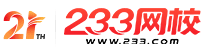#计算机二级

•扫描二维码进入

• 题 库扫描二维码进入

• 移动233网校移动

• 移动APP扫描二维码下载APP

## 2019年3月计算机二级MySQL强化练习及答案(18)

1.有以下程序：

#include

main()

{ int x=4，y;

do

{ y=x;

if(!y)printf("x");

else

printf("y")：

x--;

}while(x);

}

A.xyyx

B.yyyy

C.yyxx

D.yxyx

2.有以下程序：

#include

main()

{ int i=0，a=0;

while(i<20)

{ for(;;)

{if((i%10)==0)break：

else

i--;

}

i+=11：

a+=i：

}

printf("%d\n"，a);

}

A.62

B.63

C.33

D.32

3.下面程序的运行结果是( )。

#inelude

void del(char*s)

{ int i，j;

char*a;

a=s：

for(i=0，j=0;a[i]!='\0';i++)

{ if(a[i]>='0'&&a[i]<='9')

{s[j]=a[i];

j++;

}

s[j]='\0';

}

}

main()

{ char*s="aa89gggh";

del(s)：

printf("\n%s"，s);

}

A.1

B.2

C.3

D.4

4.当输入为"hello?"时，下面程序的执行结果是( )。

#include

main()

{ char c;

c=getchar();

while(c!='?')

{ putehar(e);break;

}

}

A.Rvfsu

B.h

C.?

D.hello

5.有以下程序，其中函数f的功能是将多个字符串按字典顺序排序( )。

#include

void f(char*p[]，int n)

{ char*t;int i，j;

for(i=0;i<N-1;I++)< p>

for(j=i+1;j<N;J++)< p>

if(strcmp(p[i]，p[j]>0){t=p[i];p[i]=p[j];p[j]

=t;}

}

main()

{ char *P={"abe"，"aabdfg"，"abbd"，"dcd-

be"，"cd"};

f(p，5);

printf("%d\n"，strlen(p));

}

A.2

B.3

C.6

D.4

6.在下列语句中，其含义为“q是一个函数指针，该指针指向整型数据”的定义语句是( )。

A.int**q;

B.int(*q)();

C.int*q;

D.int*q();

7.若输入“abcdef”“abdef”，下述程序的输出结果为( )。

#include

#include

main()

{ int n;

char s1，s2，*p1，*p2;

scanf("%s"，s1);

scanf("%S"，s2);

p1=s1;

p2=s2;

n=strcmp(p1，p2);

printf("Vood\n"，n);

}

A.-1

B.0

C."abcdef"

D."abdef"

8.下述程序用来输出两个字符串前7个字符中对应相等的字符及其位置号，则程序空白上应该填写的是( )。

#include

main()

{ char s1[]="chinese"，s2[]="japanese";

int i;

for(i=0;s1[i]='\0'&&s2[i]!='\0';i++)

if(s1[i]==s2[i]&&i<7)

_____________;

}

A.putchar(s2[i]);putchar(i)

B.puts(s1[i]，i)

C.printf("%c%d\n"，s2[i]。i)

D.printf("%C"，s1[]);printf("%d\n"，i)

9.若有以下程序：

int a=1，b=2：

a=a^b：

b=b^a：

A.a=1，b=2

B.a=3，b=l

C.a=3，b=2

D.a=2.b=1

10.利用fseek函数可实现的操作是( )。

A.实现文件的顺序读写

B.改变文件的位置指针

C.实现文件的随机读写

D.以上答案均正确

• 海量免费模拟试题，在线测试。

• 各科每年真题试卷，实战演练！

• 逐章逐节针对练习，专项突破。

• 每天10道最新试题，随机练习。

• QQ群号：806931213

扫描二维码加群• ##### 微信订阅号：kszx233

扫描二维码加群